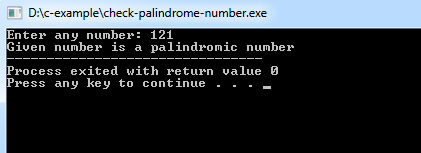# C Program to Check whether the Given Number is a Palindromic

If a number, which when read in both forward and backward way is same, then such a number is called a palindrome number.

Example:
121, 23532, 12321 etc.

## Palindrome Number Check Program in C

Program:
``````#include <stdio.h>

int main() {
int n, n1, rev = 0, rem;

printf("Enter any number: ");
scanf("%d", &n);
n1 = n;

/* logic */    while (n > 0){
rem = n % 10;
rev = rev * 10 + rem;
n = n / 10;
}

if (n1 == rev){
printf("Given number is a palindromic number");
}
else{
printf("Given number is not a palindromic number");
}
return 0;
}
``````
Program Output:Explanation:

Consider a number n=121, reverse=0 and remainder;
number=121

now the while loop is executed /* the condition (n>0) is satisfied */

/* calculate remainder */
remainder of 121 divided by 10=(121%10)=1;
now reverse=(reverse*10)+remainder
=(0*10)+1 /* we have initialized reverse=0 */
=1
number=number/10
=121/10
=12

now the number is 12, greater than 0. The above process is repeated for number=12.
remainder=12%10=2;
reverse=(1*10)+2=12;
number=12/10=1;
now the number is 1, greater than 0. The above process is repeated for number=1.
remainder=1%10=1;
reverse=(12*10)+1=121;
number=1/10 /* the condition n>0 is not satisfied,control leaves the while loop */

Program stops here. The given number=121 equals the reverse of the number. Thus the given number is a palindrome number.